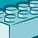# New to Qlik Sense

If you’re new to Qlik Sense, start with this Discussion Board and get up-to-speed quickly.

Announcements
Our next Qlik Insider session will cover new key capabilities. Join us August 11th REGISTER TODAY
cancel
Showing results for
Did you mean:Contributor III

## Qlik sense bar chart

I have bar chart. My dimension is Year. Measures

`=Sum({\$<Year=,Date = {">=\$(=YearStart(Max(date)))<=\$(=MonthEnd(Max(date)))"}>} FactSoldAmount) --- for the year 2018=Sum({\$<Year=,Date = {">=\$(=YearStart(AddYears(Max(date), -1)))<=\$(=MonthEnd(AddYears(Max(date), -1)))"}, BranchID = p({<Year = {"\$(=Max(Year))"}>})>} FactSoldAmount) --- for the year 2017=Sum({\$<Year=,Date = {">=\$(=YearStart(AddYears(Max(date), -2)))<=\$(=MonthEnd(AddYears(Max(date), -2)))"}, BranchID = p({<Year = {"\$(=Max(Year))"}>})>}FactSoldAmount) ----for the year 2016`

When I select year, these formulas show me only the selected years and previous years. And I want to show all of the years. For example: If I select 2017, in the bar chart I should the comparison of the years 2016, 2017, and 2018. Now, when I select 2017 it shows me only the year 2016 and 2017.

Is it possible?

2 RepliesMVP

Try with using 1 inside Element functions

BranchID = p({1<Year = {"\$(=Max(Year))"}>})Contributor III
Author

It shows the sameTags
Community Browser